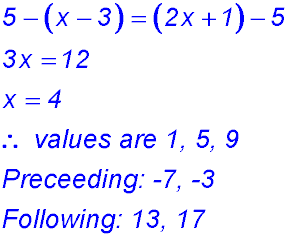Dr. J's Maths.com
Where the techniques of Maths
are explained in simple terms.

Sequences & Series - Arithmetic - the General term.
Test Yourself 2 - Solutions.

Answer. T1 = 2 and d = 3.
 Finding a term 1. The arithmetic sequence 7, 11 and 15: So T1 = 7 and d = 4. For 111 to be a member of this sequence: 111 = 7 + (n-1)×4 108 = 4n n = 27 which is an integer. ∴ 111 is a member of the sequence. 2. Series is 84, 78 and 72 So T1 = 84 and d = -6 ∴-36 = 84 + (n - 1)(-6) 6n = 126 n = 21 - which is an integer. So -36 is part of the series and is the 21st term. 3. Sn = 25n - 2n2. (i) The 2nd term = S2 - S1 S2 = 42 and S1 = 23 - so T2 = 19. (ii) Tn = Sn - Sn-1 Sn = 25n - 2n2 and Sn-1 = 25(n-1) - 2(n-1)2 ∴25n - 2n2 - (25n - 25 - 2n2 + 2n - 2) = 27 - 4n (iii) First term less than -500. We know that the series starts 23 + 19 - so the difference = -4 -500 = 23 + (n-1)(-4) 4n = 527 n = 131.75 - so n = 132 Substituting: T132 = 23 + 131×(-4) = -501 So -501 is the 132 term and it is the first term less than -500. Finding a 1st term 4. 5. Finding a difference. 6.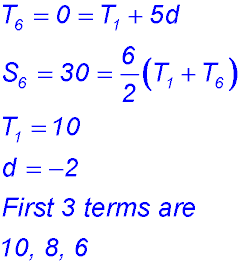7. Finding the number of terms 10. (i) Sum of interior angles = (n - 2)×180° (ii) T1 = 120° and d = 5°.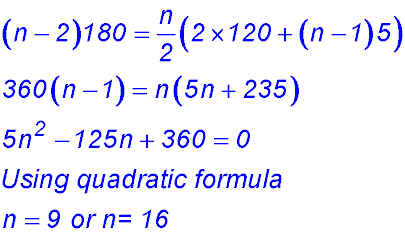11.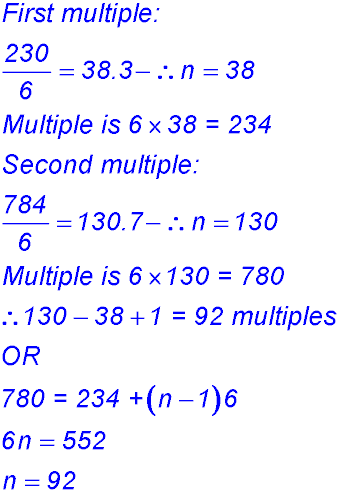12. Given 2 terms 13.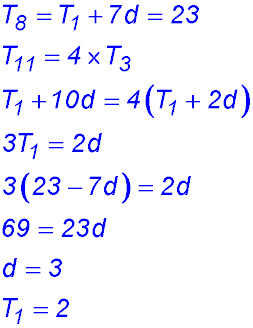14. Answer. 15. Miscellaneous. 17.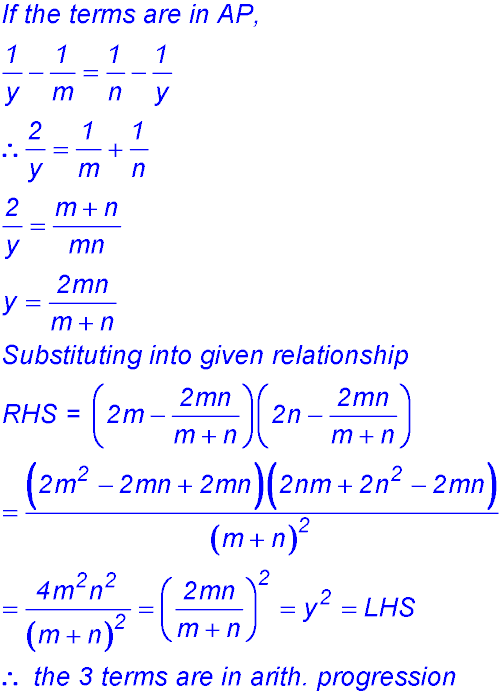18.19.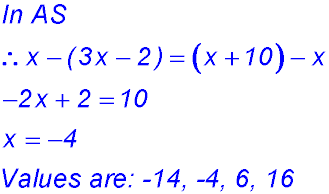20.